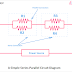## What is a Series-Parallel Circuit?

Series-Parallel Circuit is a type of electrical or electronic circuit that is a combination of a Series Circuit and a Parallel Circuit. A series-parallel Circuit is a more complex circuit than an individual series or parallel circuit. A series-parallel circuit may be built with multiple series circuits and multiple parallel circuits. As here elements are connected in series in some parts and connected in parallel in the other parts so we cannot apply a single series circuit rule or parallel circuit rule. So, a series-parallel circuit has both characteristics of a series circuit and a parallel circuit.

## Series-Parallel Circuit Examples

Here, is the list of common examples of series-parallel circuits,
1. Bridge Rectifier Circuit
2. Audio Equalization Circuit
4. Wattmeter Internal Circuit
5. Electronic Control Circuits
6. Modulator Circuits
7. Old Fire Detector Circuits
8. Electronic Timer Circits
9. Frequency Control Circuits
10. Integrated Circuits

## Series-Parallel Circuit Diagram

Here, you can see a diagram of a simple series-parallel circuit built with some resistors.

You can see resistors R1 and R2 are connected in parallel. Same the resistors R3 and R4 are also connected in parallel. Both the parallel circuits are connected in series. The voltage across R1 and R2 will be the same as they are connected in parallel. Similarly, both R3 and R4 also have the same voltage across them. If we want to calculate the total resistance of the whole circuit then need to do calculations in two steps. First of all, calculate the resistance of individual branches using the parallel resistor formula then apply the series resistance formula.

## Series-Parallel Connection of LED

Here, we have taken an example to easily understand the concept of a series-parallel circuit.

We have six LEDs of 4V rating and a 12V battery. Now we have to connect those LEDs in such a way that all of them can glow properly without any effect.

If we connect all of them in series then we need(6*4) = 24V power source that will not work with a 12V battery. If we connect all of them in parallel, same the 12V will be applied across all the LEDs. But as the LEDs are rated 4V, they will be burned. So in this case we need to connect them in a series-parallel combination.

Here, you can see the three red LEDs are connected in series which makes the total voltage requirement 12V. On the other hand, similarly, all the three green LEDs are also connected in series which also makes the voltage requirement 12V. Now both the branches of red and green LEDs are connected in parallel.

## Applications and Uses of Series-Parallel Circuit

You will see the applications of a series-parallel circuit in most of the electronic circuits which are built with different types of active and passive elements connected in both series and parallel combinations. You can see the example section above. These circuits are used with the aim of voltage, current divisions, function, features, etc.

Series-Parallel Circuit Examples, Diagram, ApplicationsReviewed by Author on July 01, 2022 Rating: 5